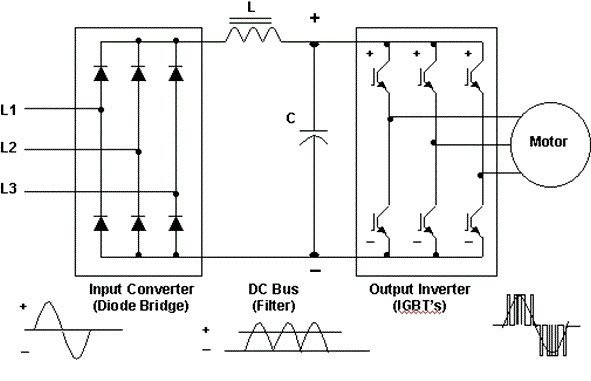# How frequency inverter works?

The frequency inverter has great importance in electric cars and renewable energy generation. This circuit operation is today’s subject.

### Simplified circuit

The inverter transforms continuous voltage, from electric car’s batteries or photovoltaic solar panels, in alternate voltage with a sinusoidal or square signal. To understand the operation, let’s first considerate this simplified circuit which reminds a H-bridge.

In circuit above, when S1 and S4 are closed while S2 and S3 are opened, current flows in direction shown.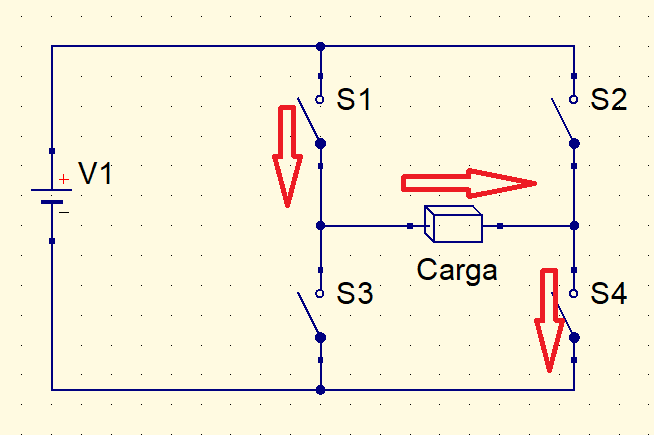When key states are inverted, current flows in opposite direction.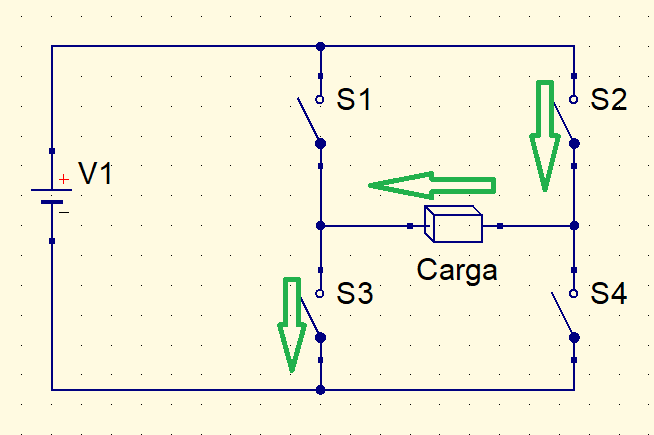With keys opening and closing in a frequency, a square wave is produced in the load.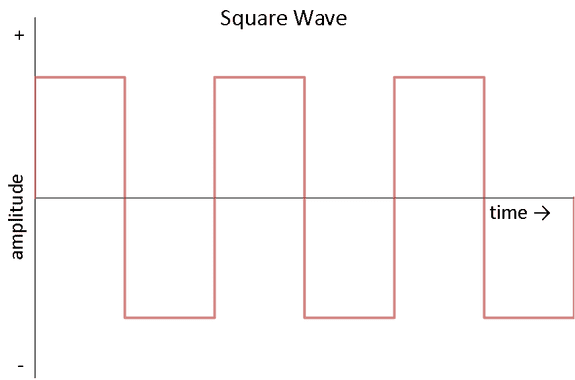To have a frequency of 50 or 60 Hz, keys must switch 100 or 120 times per second. This speed can only be obtained with semiconductors, like MOSFETs or IGBTs transistors. These components control current flow receiving control signals in gate terminal. More details will be subject to future posts.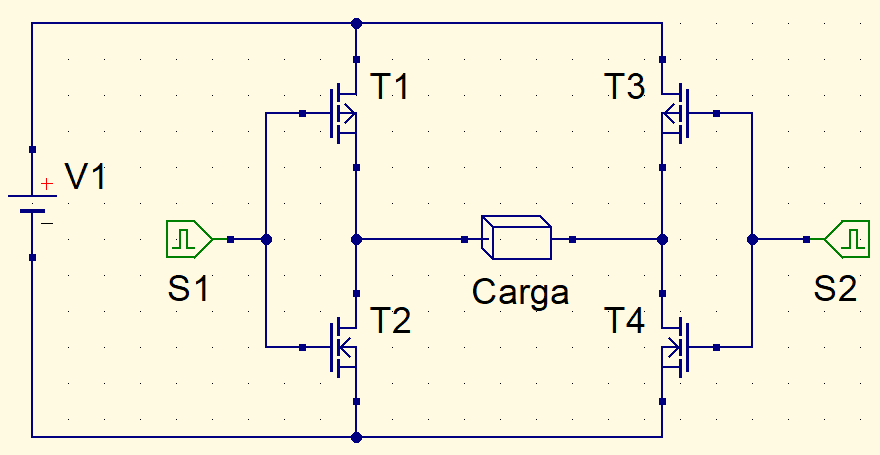T1 and T3 transistors must be of different types from T2 and T4 to avoid short-circuit. If keys are IGBTs, must use a logic gate NOT in T1 or T2 and another in T3 or T4.

### Square wave inverter

Many equipment produce a humming noise and burn a lot if they are supplied with square wave. A square wave is the sum of many sinusoids and this signal produce harmonics, waves with higher frequency, multiples of fundamental frequency.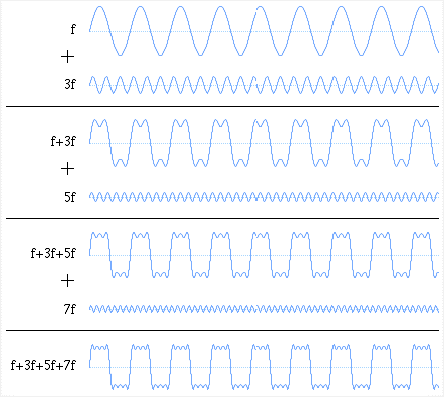The harmonics heat synchronous and induction motors and, can damage electronic devices. Modified sinusoidal wave (MSW) inverter produces a square wave as shown below.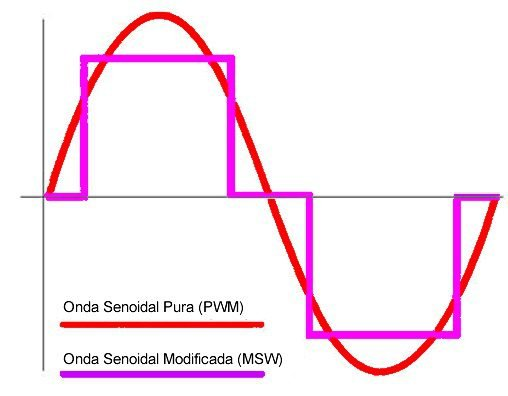MSW inverters are cheaper and can be used in illumination without involving LEDs, devices which use resistance for heating and chargers.

### Producing sinusoidal wave

How inverter produces a pure sinusoidal wave? A control circuit uses Pulse Width Modulation (PWM) technique, transmits pulses of different widths to transistors gate terminal.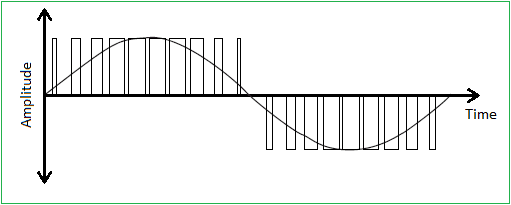Pulses with larger width are generated where needs higher amplitude. If get all average voltages from each pulse, the resulting signal will be similar to a sinusoid. When higher frequency of PWM signal, bigger the similarity with sinusoidal wave.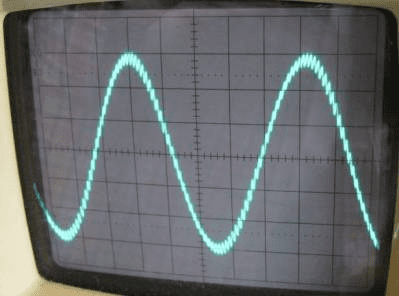How is PWM signal generated? Oscillator circuits produce a sinusoid and a triangular signal, both signals go to a comparator circuit (OP1 and OP2). Sine waves of OP1 and OP2 are offset by 180º to each other.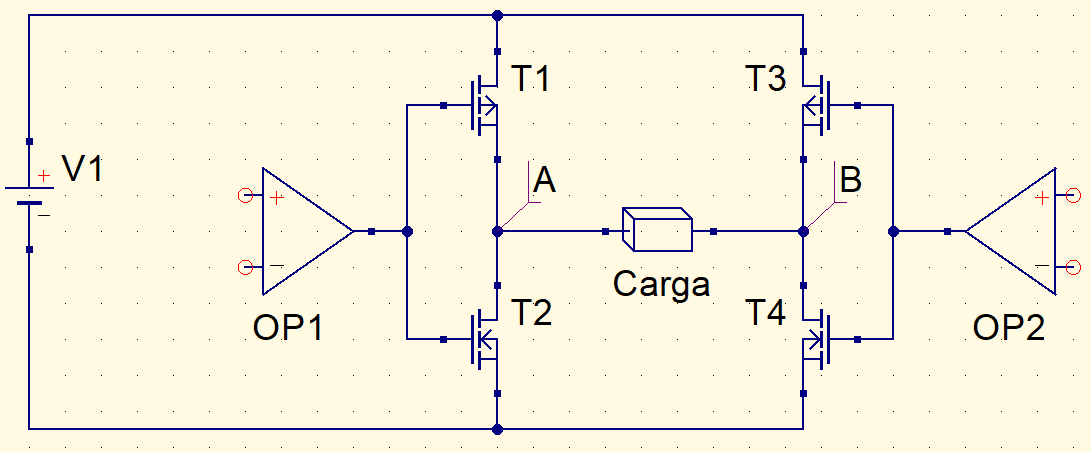If sinusoid’s amplitude is higher than triangular signal, comparator’s output will be voltage level 1 or high, otherwise, will be 0 or low.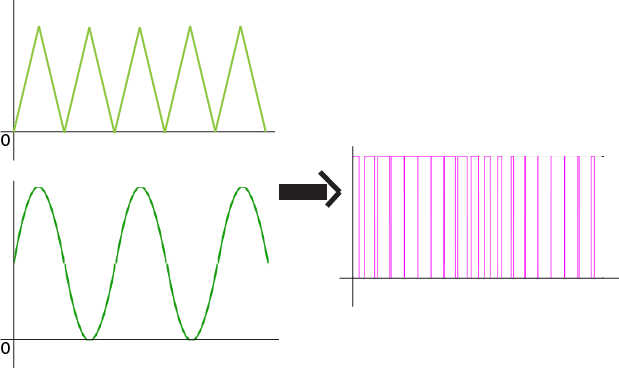Combining signals in points A and B, we have PWM shown before.Inverters have transformers in output to elevate voltage, allowing to supply home appliances.

### Inverter for three phase motors

Inverters for electric motors also are called VFD (Variable Frequency Driver). Have a diode rectifier bridge to convert three phase voltage in direct. Also have a LC filter and a three phases IGBT bridge to control induction motor speed.